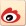## 探寻中国经济未来一个阶段的合意增长速度

Y=AKαLβ

ln(Y)=ln(A)+αln(K)+βln(L)

ln(Y/L)=ln(A)+αln(K/L)

Kt=Kt-1(1-dt)+It（一）政治层面要求：实现全面建成小康社会的总体目标

（二）经济社会发展潜力：基于新型城镇化战略的考察（三）中国发展中面临的约束：债务压力和环境压力

ln(GDP/L)t=c0+at(k)ln(K/L)t+at(CO2)ln(CO2/L)t+att1960-2012+ut(C0)

at(k)=at(k)(-1)，at(CO2)=at(CO2)(-1)，at=at(-1)

（作者刘陈杰为望正资本全球宏观对冲基金董事长、李晓磊为华德资本集团有限公司总经理；编辑：苏琦）

（本文首刊于2019年5月13日出版的《财经》杂志）(编辑：陈颖)• 热文
• 本周热文
• 热图
• 热评
• 博客
• 财讯集团
• 合作伙伴
• 财经资讯
• 股票金融
• 商务管理
• 时尚娱乐
• 友情视频
• 友情链接